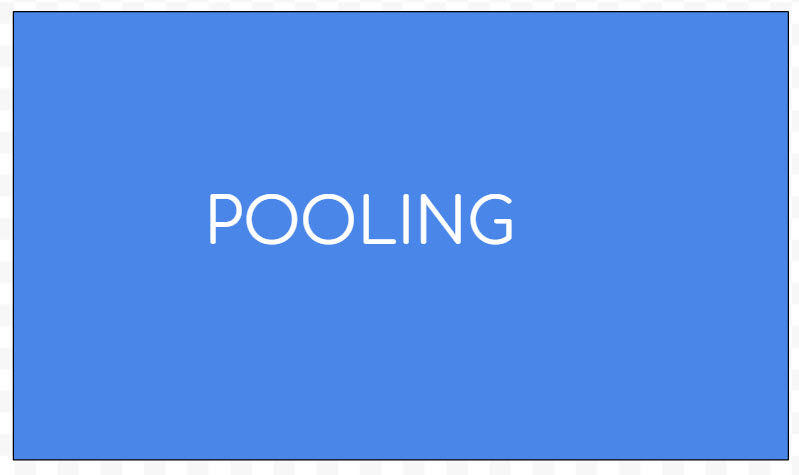top of pageSearch

# Why Pooling in CNN?

Imagine you are scanning a 16X20 picture and a 2X2 same picture, which one do you think is scanned faster? Yes 2X2 would be faster with less computational power. This downsizing to process fast is called Pooling. Let us see more details about Pooling.In Deep learning Convolutional neural networks(CNN) is a class which is used to analyze data which depends on the sense of sight like image recognition, video recognition, image classification, medical image analysis etc.

It consists of input layer, hidden layers and output layer. The hidden layers can be multiple convolved layers. These layers convolve the input and pass the result to the next layer and the featured image is abstracted. A concern with the output feature maps is that they are sensitive to the location of the features in the input. Another concern is image computation cost is higher as the architecture involves size of images where each pixel is different. One way to address this sensitivity and computation cost is to down size the feature maps which can be achieved by Pooling. Pooling summarizes the features in patches and thus down sizes the feature map. This in turn can control overfitting.

Hyperparameters of pooling

There are few hyper parameters used in pooling

1.filter size(f)

2.stride(s)(the length of movement of tensor which is horizontal and vertical)

The size of featured image can be calculated by using the below formulaThere are few types of pooling of which the following are popular.

Types

• Maximum Pool

• Average Pool

Let us try to understand the types with an example. Here the assumptions are the size of the image is 4*4(each cell represents each pixel).Tensor the filter size is 2*2.Stride (the length of movement of tensor which is horizontal and vertical) is 2.

Maximum Pool: This is achieved by getting the maximum value of the set of pixels. Here we are retaining the strong pixels of the image. The green pixels have value 8 as maximum and we are retaining it similarly yellow has 9,red has 9, blue has 6 as the maximum values and our max pool is achieved. Hence this down sizing helps us to overcome computation costs and overfitting.Average pooling: This is similar to max pool but instead of ignoring the lower values we are computing average and extracting the feature map. The main drawback of this way is it might not detect sharp edges or complex features. The following image shows how the feature map is extracted using Average pool technique.Conclusion

Hence pooling represents the original image which is approximately invariant to slight translations in a smaller size. This helps to reduce the computational power and also helps to overcome overfitting.